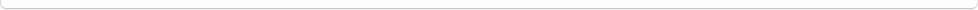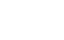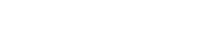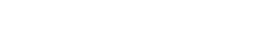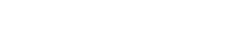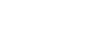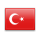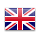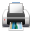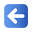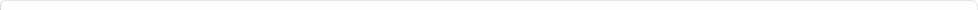Description of Individual Course Units
 Course Unit Code Course Unit Title Type of Course Unit Year of Study Semester Number of ECTS Credits 140400108111 MACROECONOMIC MODELS Elective 4 8 4
Level of Course Unit
First Cycle
Objectives of the Course
To be able to explain econometric methods for modeling economic problems and making policy recommendations based on economic theories.
Name of Lecturer(s)
Dr. Gürkan BOZMA
Learning Outcomes
 1 To be able to evaluate the problems related to macroeconomic modeling and econometric modeling. 2 To be able to explain unit root analysis, non-stationary series, cointegration and structural change. 3 To be able to do theoretical and econometric modeling of non-stationary macroeconomic time series. 4 To be able to apply the relevant macro modeling method to various fields of economics. 5 To be able to analyze the findings obtained as a result of econometric modeling in the predictions of economic theory. 6 To be able to apply scientific research rules and ethical rules on an academic study.
Mode of Delivery
Daytime Class
Prerequisites and co-requisities
Recommended Optional Programme Components
Course Contents
The course focuses on macroeconomic modeling and econometrics of macroeconomic modeling at the undergraduate level. Within this framework, non-stationary macroeconomic time series variables Emphasis is placed on the concept of econometric modeling. In this direction, the main areas of the course can be identified as problems related to macroeconomic modeling and econometric modeling, unit root analysis, non-stationary series, cointegration and structural change. The theoretical and empirical econometric modeling of non-stationary macroeconomic time series will enable those taking this course to apply the related method to various fields of economics.
Weekly Detailed Course Contents
 Week Theoretical Practice Laboratory 1 Basic Concepts: Model, Modeling, Economic Model, Mathematical Model, Econometric Model, Economic Theory and empiricism, Modeling Requirement 2 Exercise: Fundamental Keynesian Model for the Small Open Economy 3 Econometric Modeling (Estimation, Forecasting and Simulation Methods), univariate, single equation and multi equilibrium (system) modeling and estimation 4 Time Series, Cross Section and Panel Data Analysis 5 Time Series Econometrics and Stationarity Analysis: Theory and Practice 6 Data Analysis, Stationarity of the series, spurious regression, unit root 7 Long-run, Economic Theory and Cointegration Analysis: Theory and Practice 8 Midtrem Week 9 Midtrem Week 10 Cointegration Analysis: Single equation and system estimation methods 11 Using Econometric models at the economic policy forecasts: Lucas Critique and structural change 12 Modeling Applications: Money Demand 13 Modeling Applications: Supply and Demand Functions of Export 14 Modeling Applications: Phillips Curve